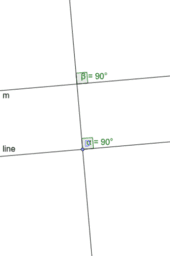# MTH 338 Homework #3

Homework 3 #6, 11, 16, 22 Equivalence to Euclid's 5th postulate.
•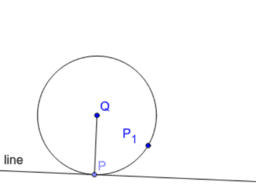### #6 PQ perpendicular to the tangent line at the point of tangency

•### #11 Prove that the perpendicular bisectors of a cyclic quadrilateral are concurrent if and only if the quadrilateral is cyclic.

•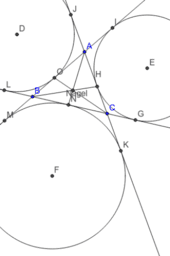### #16 The three excircles of trian...

•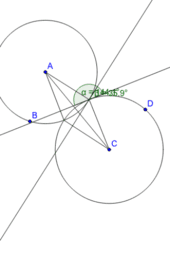### #22 Prove if two circles are orthogonal, then they intersect at exactly two points and their tangents are perpendicular at both of those points

•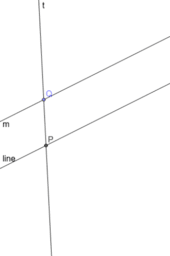### Playfair's postulate is logically equivalent to the statement: If a line intersects one of two parallel lines, then it also intersects the other

•